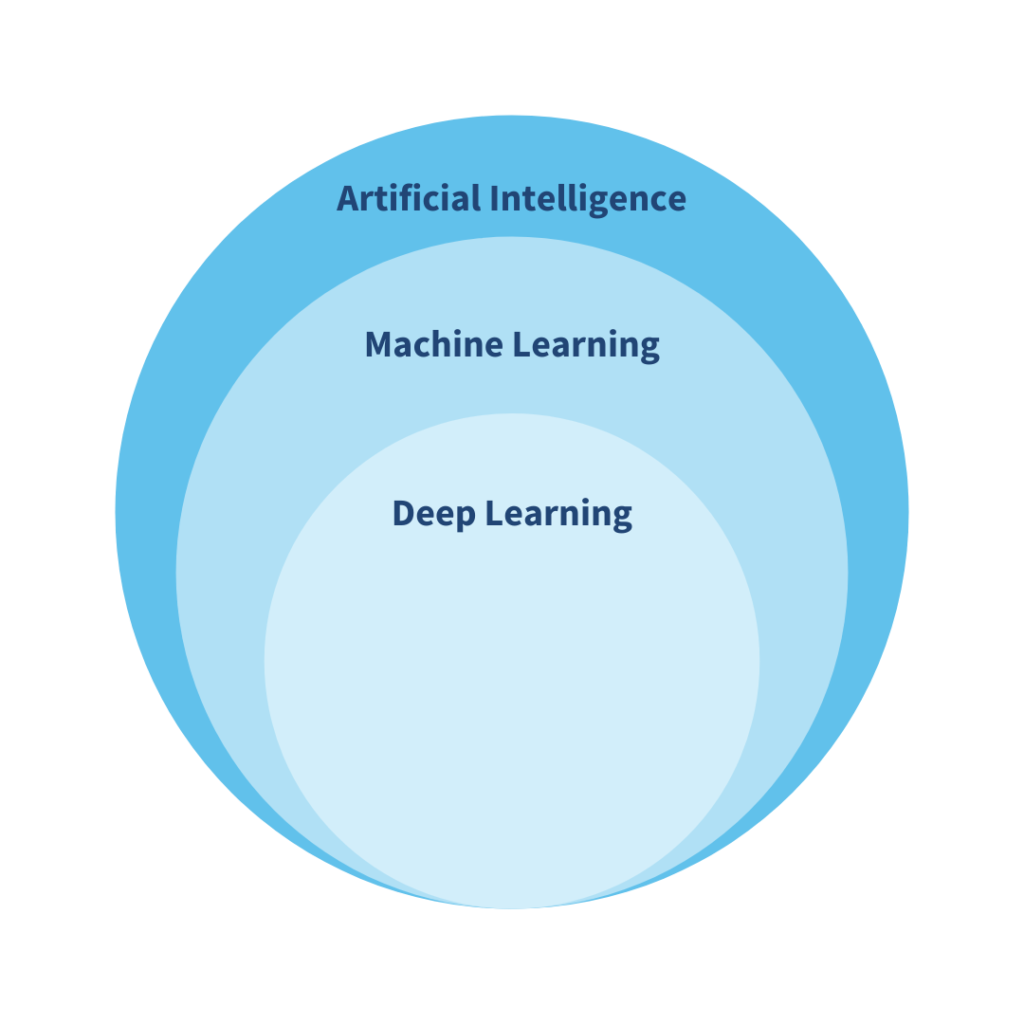Choosing the right algorithm

Artificial Intelligence (AI) helps a computer to mimic humans with the help of Machine Learning (ML) algorithms. ML is the branch of AI that enables the machine to make predictions or classifications based on the data it has been trained on. Deep learning (DL) is the subset of machine learning that uses neural networks, works like the neurons of our brain, that makes intelligent decisions on its own.For the last few decades, many applications of AI have been developed in different areas such as fraud detection, tumour detection, weather forecasting, stock prediction, object detection, robotics etc. Many more are in developing stage such as self-driving cars which will continue to be refined in the next few years. The existing AI models are working perfectly fine with good accuracy. Hence, it is very important to choose right algorithms to get an accurate model.

ML has variety of algorithms that are suitable for small datasets and take less time to train the model. However, DL algorithms such as RNN (Recurrent Neural Networks), CNN (Convolutional Network Network) etc. are suitable for large datasets and complex applications and take a large time to train the model. Performance for the ML/DL model depends on the size and dimensionality of the dataset, capacity to handle the outliers, ability of hyperparameter tuning, and computational speed. There is always a confusion that DL models gives higher accuracy than the ML models. It is not in every case as performance of the model depends upon the size and type of the dataset and neural networks need large amount of data to train effectively. If the dataset is small, there may be the chances that DL model will not perform well.

In the ocean sectors there are various application such as fish weight prediction, fish classification, fish detection, and weather prediction where we could apply AI models. Prediction of the fish weight is a regression problem which can be solved with algorithms such as linear regression or polynomial regression. Fish Detection and Classification is the classification problem. In the latter case, the dataset will be images and require an algorithm like CNN for better accuracy. For recurrent problems, RNN can be used, e.g. for the prediction of weather based on historical weather data. Ocean data can be huge and the DL models can be very complex. Consequently, training the models on a huge dataset requires huge computational power. DeepSense’s High-Performance Computing (HPC) infrastructure provides huge memory, multi-cores, high-end GPU’s, and huge storage to accelerate the computation and thus greatly improve the efficiency and accuracy of real-life applications.